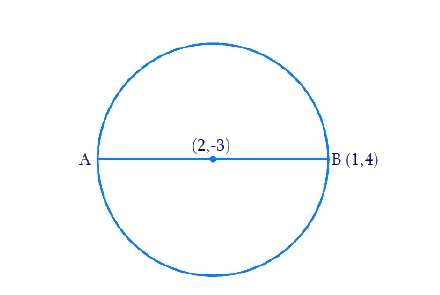# Ex.7.2 Q7 Coordinate Geometry Solution - NCERT Maths Class 10

## Question

Find the coordinates of a point $$A$$, where $$AB$$ is the diameter of circle whose center is $$(2, -3)$$ and $$B$$ is $$(1, 4)$$

Video Solution
Coordinate Geometry
Ex 7.2 | Question 7

## Text Solution

Reasoning:

The coordinates of the point $$P(x, y)$$ which divides the line segment joining the points $$A(x_1, y_1)$$ and $$B(x_2, y_2)$$, internally, in the ratio $$m_1 : m_2$$ is given by the Section Formula.

What is the known?

The $$x$$ and $$y$$ co-ordinates of the center of the circle and one end of the diameter $$B$$.

What is the unknown?

The coordinates of a point $$A$$.

Steps:

From the Figure,Given,

• Let the coordinates of point $$A$$ be $$(x, y)$$.
• Mid-point of $$AB$$ is $$C$$ $$(2, -3)$$, which is the center of the circle.

\begin{align} \therefore \; (2, - 3) &= \left( {\frac{{x + 1}}{2},\frac{{y + 4}}{2}} \right) \end{align}

\begin{align} \Rightarrow \frac{{x + 1}}{2}& = 2\;\;{\text{ and }}\;\;\frac{{y + 4}}{2} = - 3 & & \end{align} (By Cross multiplying & transposing)

\begin{align} \Rightarrow x + 1 &= 4\;\;{\text{ and }}\;\;y + 4 = - 6 \end{align}

\begin{align} \Rightarrow x &= 3\;\;{\text{ and }}\;\;y = - 10\end{align}

Therefore, the coordinates of $$A$$ are $$(3, -10)$$

Learn from the best math teachers and top your exams

• Live one on one classroom and doubt clearing
• Practice worksheets in and after class for conceptual clarity
• Personalized curriculum to keep up with school